# multiplication Fractions Problems

#### Number of problems found: 96

• Equivalent fractionsAre these two fractions equivalent -4/9 and 11/15?
• Fractions 3Calculate 1/9 of 27:
• CompareCompare fractions ?. Which fraction of the lower?
• Write 3Write a real world problem involving the multiplication of a fraction and a whole number with a product that is between 8 and 10 then solve the problem
• Cake fractions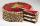Thomas ate 1/3 of cake, Bohouš of the rest of the cake ate 2/5. What fraction of cake left over for others?
• Two divided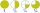Two divided by nine tenths.
• MilimetersHow many millimeters is 1/4 meters?
• Pie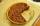Vili ate three pieces of pie . If it piece is 1/8 how much pie did he eat?
• Seeds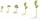Of the 72 wet seeds 7/8 germinated. How many seeds germinate?
• Classroom 4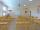In a class of 36 pupils, 2/3 are girls. How much it is in a class girls and boys?
• Women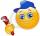In an company of 1050 employees are 2/3 women. How many women work in the company?
• EmployeesOf all 360 employees, there are 11/12 women. How many men work in a company?
• Mixed with percentagesCalculate 33 1/3% of 570.
• The machineThe machine was in operation for 9 tenths (in a fraction) of eight hours of working time. How long has he been running?
• Hours 3How many hours are in 3 2/3 days?
• Ratio - proportion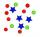Reduce the number 16 in proportion 3:2 5:4 11:8
• In centerWhat number lies in the center between a quarter of a fifth and a half of a third on the number axis?
• Babysitting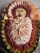\$27.40 babysitting for 4 hours. How much would she make if she babysat for 7 hours?
• Percent calculationCalculate 51% if 99% is 79.
• Two thirdsFind two-thirds of the number equal to two-thirds of 99

Do you have an interesting mathematical word problem that you can't solve it? Submit a math problem, and we can try to solve it.

We will send a solution to your e-mail address. Solved examples are also published here. Please enter the e-mail correctly and check whether you don't have a full mailbox.

Please do not submit problems from current active competitions such as Mathematical Olympiad, correspondence seminars etc...

Need help calculate sum, simplify or multiply fractions? Try our fraction calculator.